# Chemical Equations and Reactions Chapter 8 Describing Chemical

• Slides: 73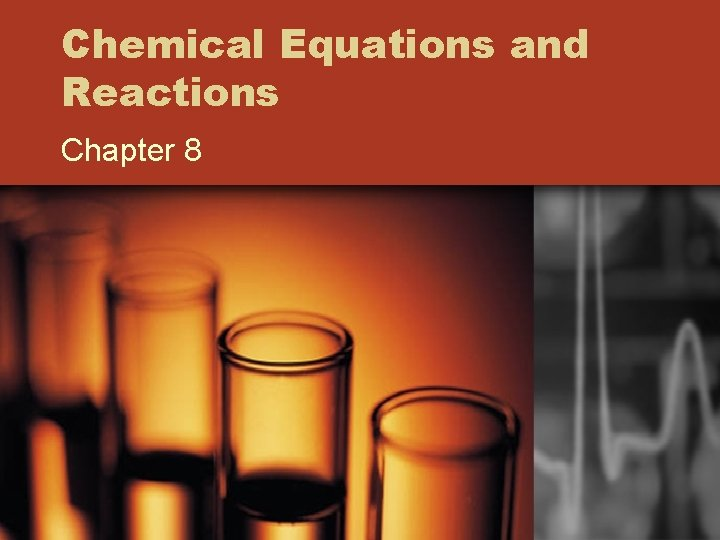Chemical Equations and Reactions Chapter 8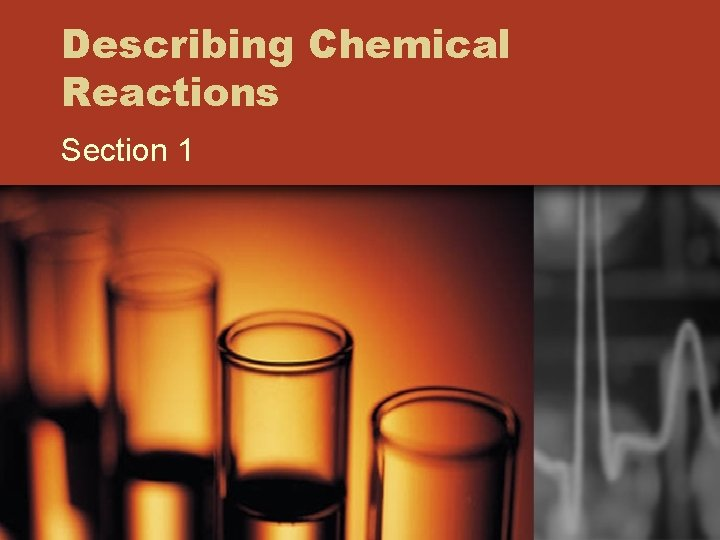Describing Chemical Reactions Section 1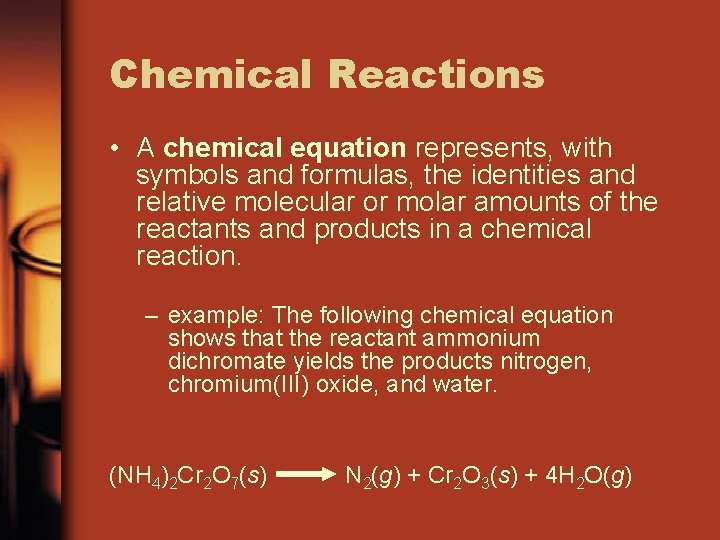Chemical Reactions • A chemical equation represents, with symbols and formulas, the identities and relative molecular or molar amounts of the reactants and products in a chemical reaction. – example: The following chemical equation shows that the reactant ammonium dichromate yields the products nitrogen, chromium(III) oxide, and water. (NH 4)2 Cr 2 O 7(s) N 2(g) + Cr 2 O 3(s) + 4 H 2 O(g)Indications of a Chemical Reaction • Certain easily observed changes usually indicate that a chemical reaction has occurred. 1. Evolution of energy as heat and light - Magnesium example 2. Production of a gas - Bubbles – Baking soda and vinegar – CO 2 3. Formation of a precipitate. • A solid that is produced as a result of a chemical reaction in solution and that separates from the solution is known as a precipitate. 4. Color changeCharacteristics of Chemical Equations • The following requirements will aid you in writing and reading chemical equations correctly. 1. The equation must represent known facts. 2. The equation must contain the correct formulas for the reactants and products. 3. The law of conservation of mass must be satisfied. • A coefficient is a small whole number that appears in front of a formula in a chemical equation.Elements That Normally Exist as Diatomic MoleculesWord and Formula Equations • The first step in writing a chemical equation is to identify the facts to be represented. • A word equation is an equation in which the reactants and products in a chemical reaction are represented by words. – A word equation is qualitative • example: methane + oxygen carbon dioxide + water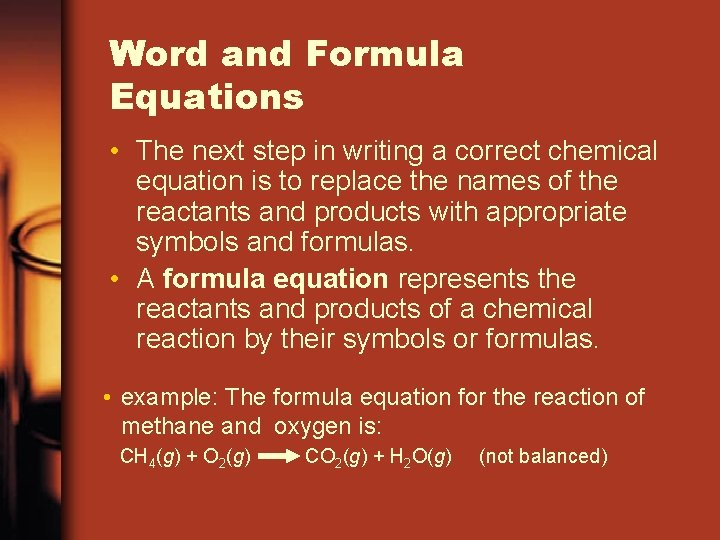Word and Formula Equations • The next step in writing a correct chemical equation is to replace the names of the reactants and products with appropriate symbols and formulas. • A formula equation represents the reactants and products of a chemical reaction by their symbols or formulas. • example: The formula equation for the reaction of methane and oxygen is: CH 4(g) + O 2(g) CO 2(g) + H 2 O(g) (not balanced)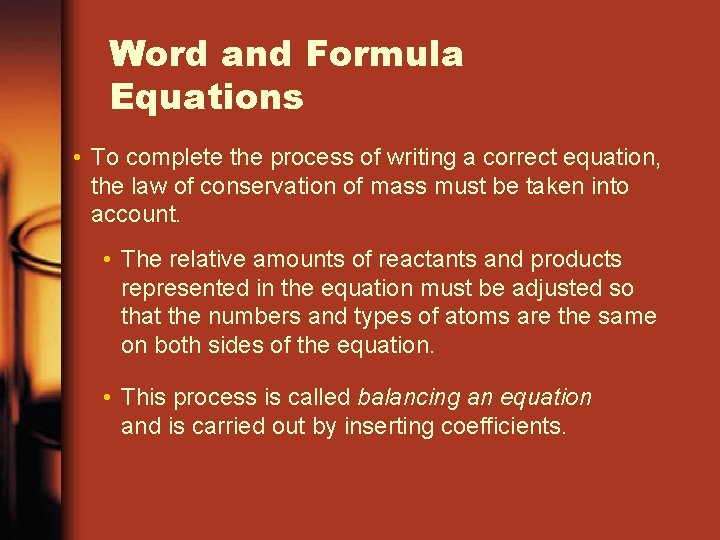Word and Formula Equations • To complete the process of writing a correct equation, the law of conservation of mass must be taken into account. • The relative amounts of reactants and products represented in the equation must be adjusted so that the numbers and types of atoms are the same on both sides of the equation. • This process is called balancing an equation and is carried out by inserting coefficients.Word and Formula Equations • To balance the equation, begin by counting atoms of elements that are combined with atoms of other elements and that appear only once on each side of the equation. CH 4(g) + O 2(g) CO 2(g) + 2 H 2 O(g) (not balanced) • Begin by counting carbon atoms. • Carbon is already balanced in the equation. • Two additional hydrogen atoms are needed on the right side of the equation.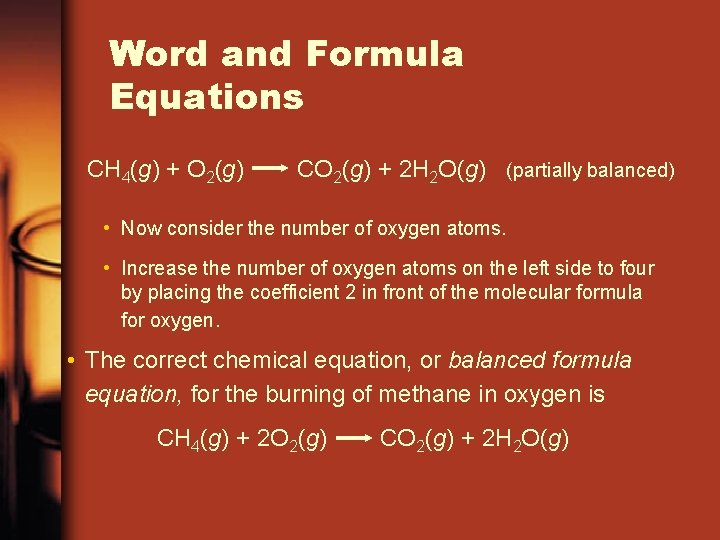Word and Formula Equations CH 4(g) + O 2(g) CO 2(g) + 2 H 2 O(g) (partially balanced) • Now consider the number of oxygen atoms. • Increase the number of oxygen atoms on the left side to four by placing the coefficient 2 in front of the molecular formula for oxygen. • The correct chemical equation, or balanced formula equation, for the burning of methane in oxygen is CH 4(g) + 2 O 2(g) CO 2(g) + 2 H 2 O(g)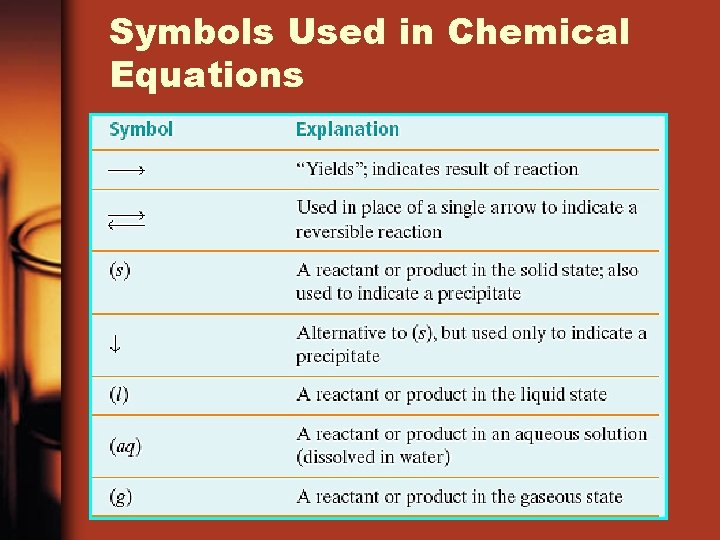Symbols Used in Chemical Equations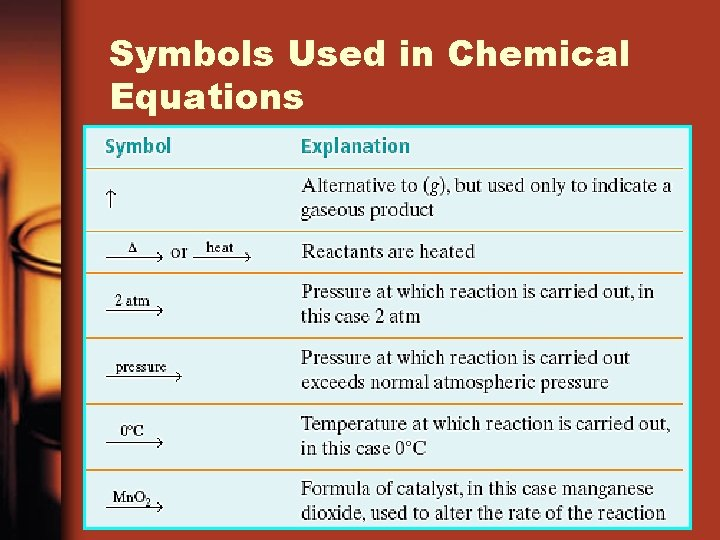Symbols Used in Chemical EquationsSymbols Used in Chemical Equations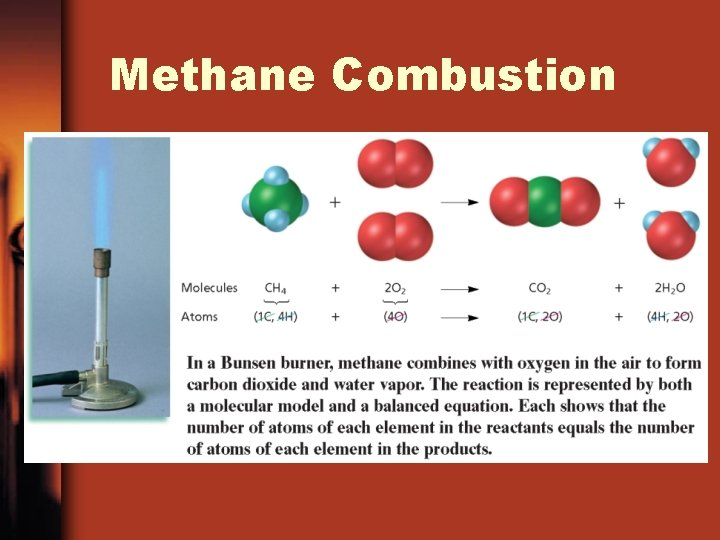Methane CombustionExample • Write word and formula equations for the chemical reaction that occurs when solid sodium oxide is added to water at room temperature and forms sodium hydroxide (dissolved in the water). Include symbols for physical states in the formula equation. Then balance the formula equation to give a balanced chemical equation.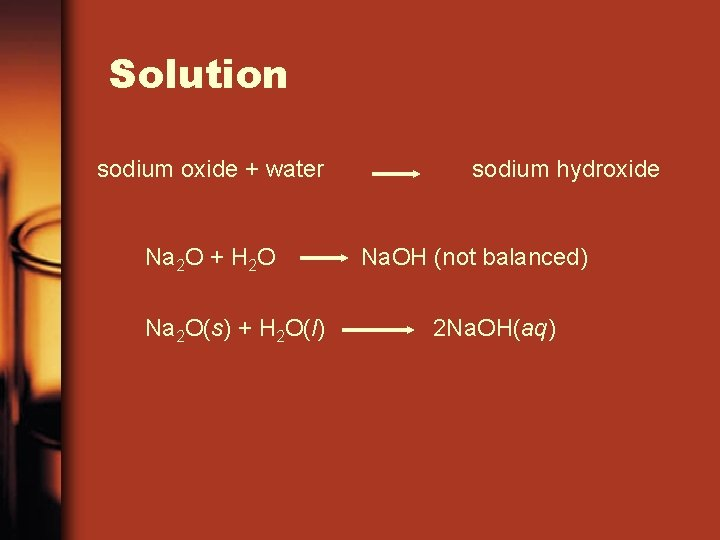Solution sodium oxide + water Na 2 O + H 2 O Na 2 O(s) + H 2 O(l) sodium hydroxide Na. OH (not balanced) 2 Na. OH(aq)Example II Translate the following chemical equation into a sentence: Ba. Cl 2(aq) + Na 2 Cr. O 4(aq) Ba. Cr. O 4(s) + 2 Na. Cl(aq) Aqueous solutions of barium chloride and sodium chromate react to produce a precipitate of barium chromate plus sodium chloride in aqueous solution.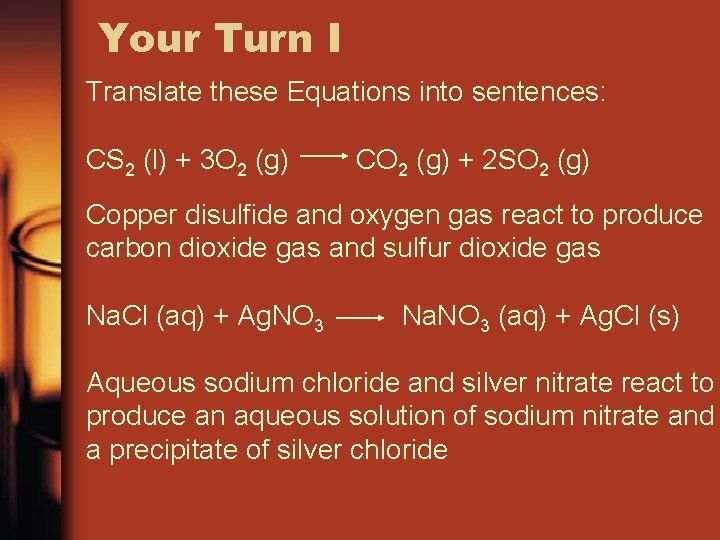Your Turn I Translate these Equations into sentences: CS 2 (l) + 3 O 2 (g) CO 2 (g) + 2 SO 2 (g) Copper disulfide and oxygen gas react to produce carbon dioxide gas and sulfur dioxide gas Na. Cl (aq) + Ag. NO 3 Na. NO 3 (aq) + Ag. Cl (s) Aqueous sodium chloride and silver nitrate react to produce an aqueous solution of sodium nitrate and a precipitate of silver chlorideSignificance of a Chemical Equation • Some of the quantitative information revealed by a chemical equation includes 1. The coefficients of a chemical reaction indicate relative, not absolute, amounts of reactants and products. H 2(g) + Cl 2(g) 2 HCl(g) 1 molecule H 2 : 1 molecule Cl 2 : 2 molecules HCl • This ratio shows the smallest possible relative amounts of the reaction’s reactants and products.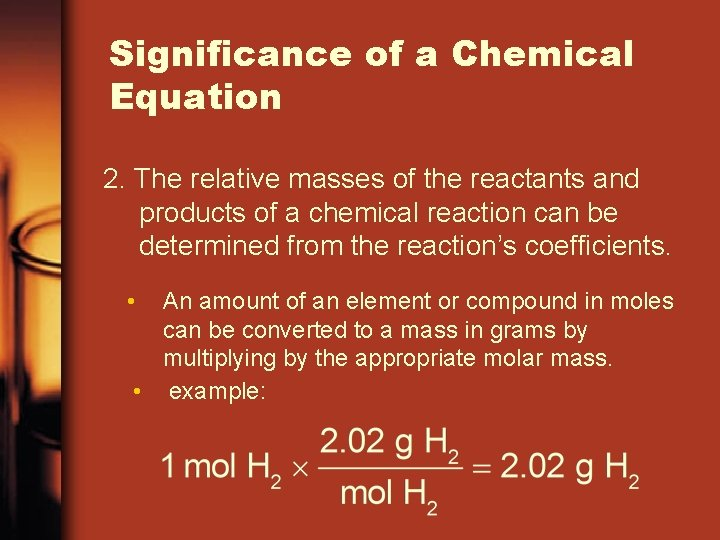Significance of a Chemical Equation 2. The relative masses of the reactants and products of a chemical reaction can be determined from the reaction’s coefficients. • An amount of an element or compound in moles can be converted to a mass in grams by multiplying by the appropriate molar mass. • example:Interpreting a Chemical ReactionSignificance of a Chemical Equation 3. The reverse reaction for a chemical equation has the same relative amounts of substances as the forward reaction. • An equation gives no indication of whether a reaction will actually occur. • Chemical equations give no information about the speed at which reactions occur. • Equations do not give any information about how the bonding between atoms or ions changes during the reaction.Visual Concept • EquationBalancing Chemical Equations • The following procedure demonstrates how to master balancing equations by inspection using a step-bystep approach. 1. Identify the names of the reactants and the products, and write a word equation. water hydrogen + oxygenBalancing Chemical Equations 2. Write a formula equation by substituting correct formulas for the names of the reactants and the products. H 2 O(l) H 2(g) + O 2(g) (not balanced)Balancing Chemical Equations 3. Balance the formula equation according to the law of conservation of mass. • Balance the different types of atoms one at a time. • First balance the atoms of elements that are combined and that appear only once on each side of the equation. • Balance polyatomic ions that appear on both sides of the equation as single units. • Balance H atoms and O atoms after atoms of all other elements have been balanced.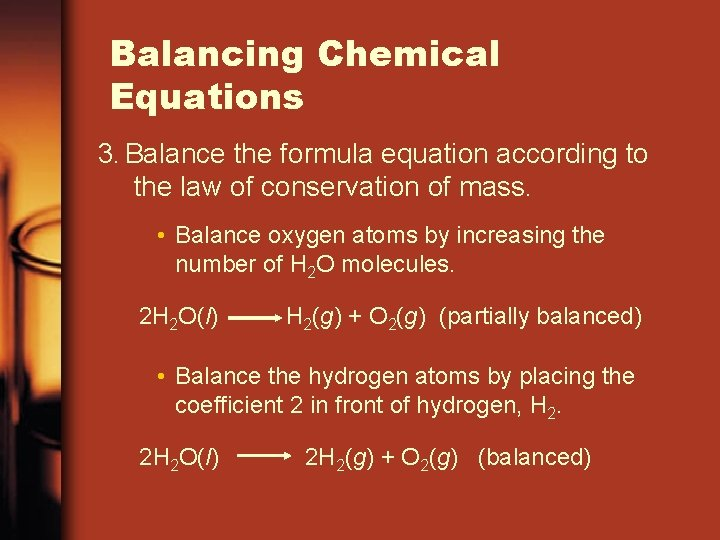Balancing Chemical Equations 3. Balance the formula equation according to the law of conservation of mass. • Balance oxygen atoms by increasing the number of H 2 O molecules. 2 H 2 O(l) H 2(g) + O 2(g) (partially balanced) • Balance the hydrogen atoms by placing the coefficient 2 in front of hydrogen, H 2. 2 H 2 O(l) 2 H 2(g) + O 2(g) (balanced)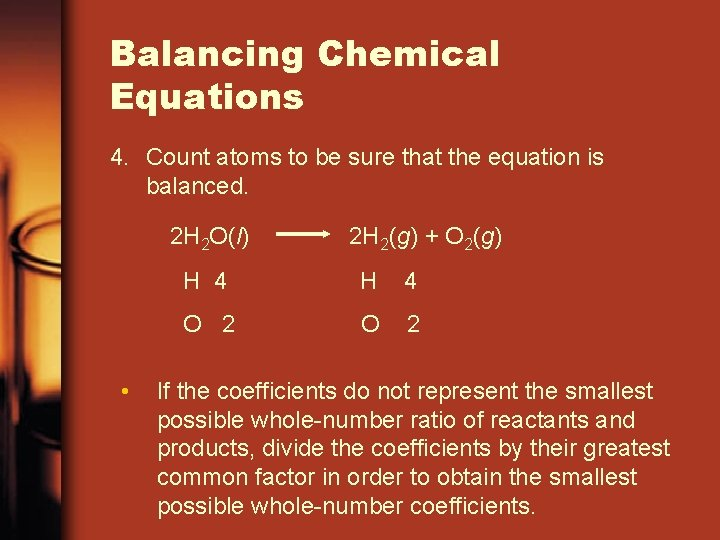Balancing Chemical Equations 4. Count atoms to be sure that the equation is balanced. 2 H 2 O(l) • 2 H 2(g) + O 2(g) H 4 O 2 If the coefficients do not represent the smallest possible whole-number ratio of reactants and products, divide the coefficients by their greatest common factor in order to obtain the smallest possible whole-number coefficients.Never • Change a subscript to balance an equation. • If you change the formula you are describing a different reaction. • H 2 O is a different compound than H 2 O 2 • Never put a coefficient in the middle of a formula • 2 Na. Cl is okay, Na 2 Cl is not.Visual Concept • Balancing Equations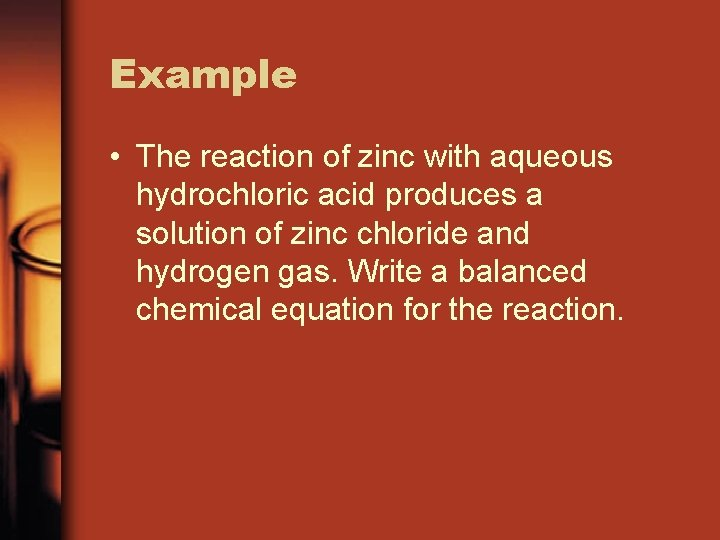Example • The reaction of zinc with aqueous hydrochloric acid produces a solution of zinc chloride and hydrogen gas. Write a balanced chemical equation for the reaction.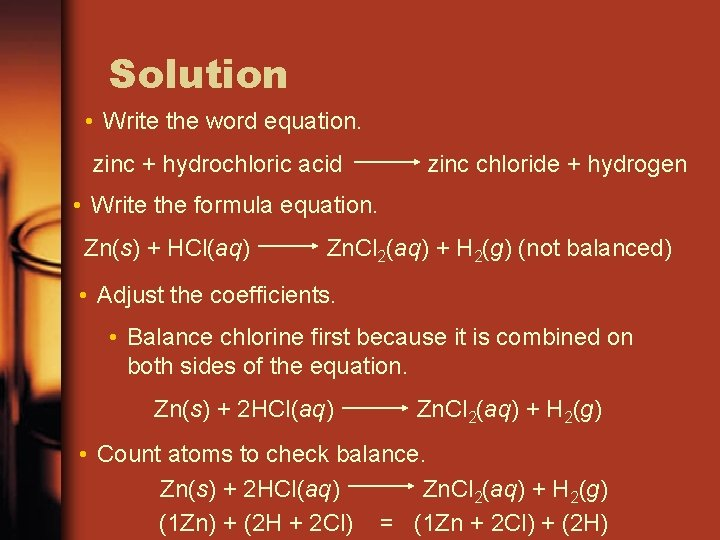Solution • Write the word equation. zinc + hydrochloric acid zinc chloride + hydrogen • Write the formula equation. Zn(s) + HCl(aq) Zn. Cl 2(aq) + H 2(g) (not balanced) • Adjust the coefficients. • Balance chlorine first because it is combined on both sides of the equation. Zn(s) + 2 HCl(aq) Zn. Cl 2(aq) + H 2(g) • Count atoms to check balance. Zn(s) + 2 HCl(aq) Zn. Cl 2(aq) + H 2(g) (1 Zn) + (2 H + 2 Cl) = (1 Zn + 2 Cl) + (2 H)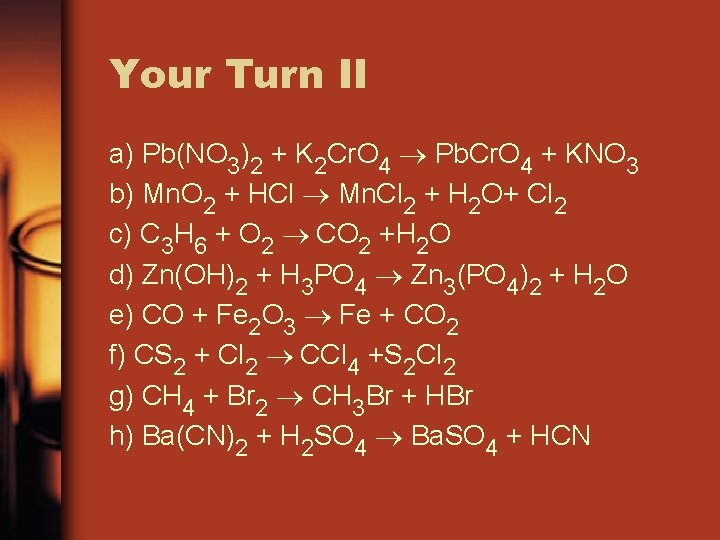Your Turn II a) Pb(NO 3)2 + K 2 Cr. O 4 Pb. Cr. O 4 + KNO 3 b) Mn. O 2 + HCl Mn. Cl 2 + H 2 O+ Cl 2 c) C 3 H 6 + O 2 CO 2 +H 2 O d) Zn(OH)2 + H 3 PO 4 Zn 3(PO 4)2 + H 2 O e) CO + Fe 2 O 3 Fe + CO 2 f) CS 2 + Cl 2 CCl 4 +S 2 Cl 2 g) CH 4 + Br 2 CH 3 Br + HBr h) Ba(CN)2 + H 2 SO 4 Ba. SO 4 + HCN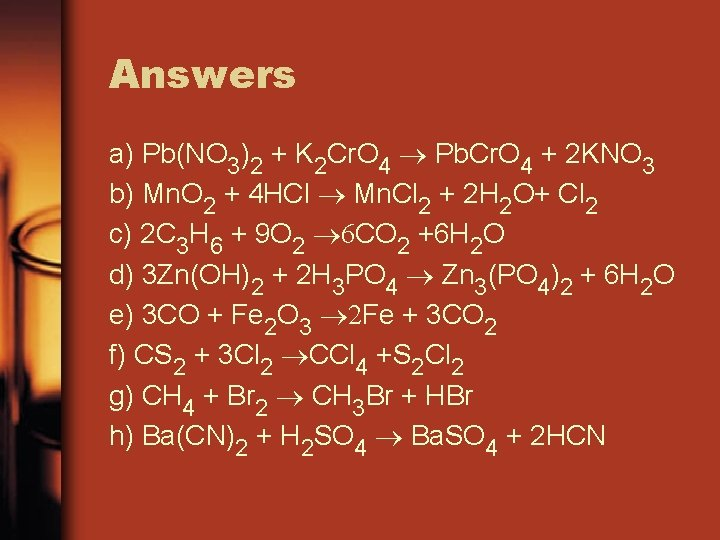Answers a) Pb(NO 3)2 + K 2 Cr. O 4 Pb. Cr. O 4 + 2 KNO 3 b) Mn. O 2 + 4 HCl Mn. Cl 2 + 2 H 2 O+ Cl 2 c) 2 C 3 H 6 + 9 O 2 6 CO 2 +6 H 2 O d) 3 Zn(OH)2 + 2 H 3 PO 4 Zn 3(PO 4)2 + 6 H 2 O e) 3 CO + Fe 2 O 3 2 Fe + 3 CO 2 f) CS 2 + 3 Cl 2 CCl 4 +S 2 Cl 2 g) CH 4 + Br 2 CH 3 Br + HBr h) Ba(CN)2 + H 2 SO 4 Ba. SO 4 + 2 HCNMs Houts’ Tricks • If an atom appears more than once on a side, balance it last. • If you fix everything except one element, and it is even on one side and odd on the other, double the first number, then move on from there. C 4 H 10 + O 2 CO 2 + H 2 O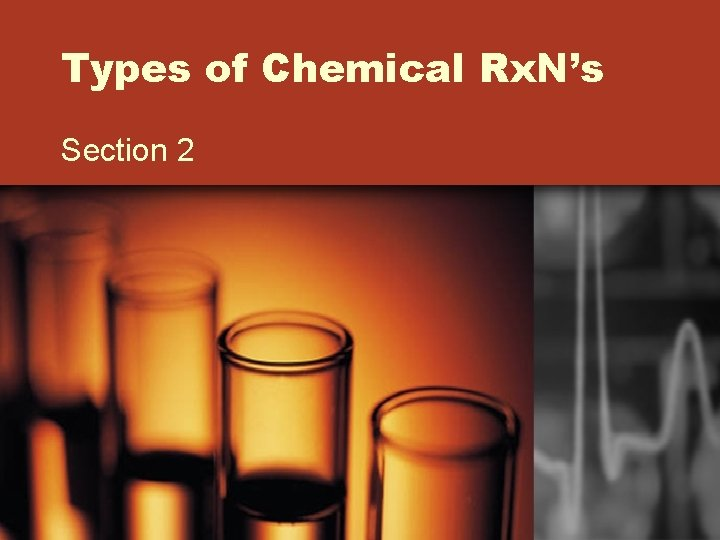Types of Chemical Rx. N’s Section 2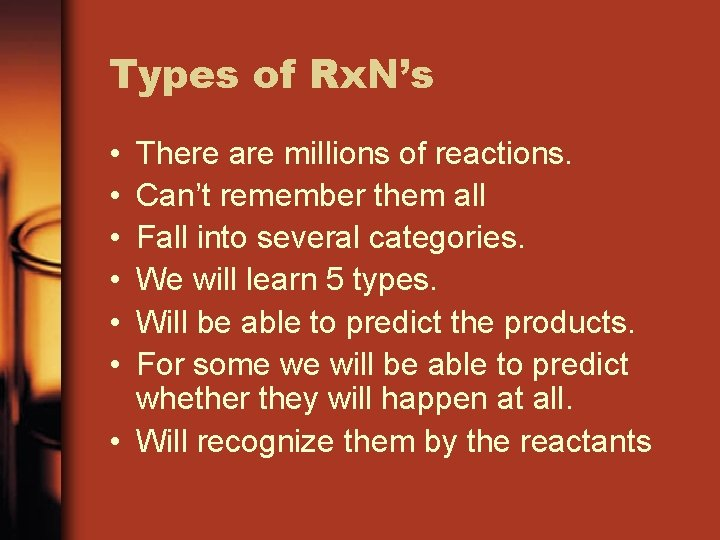Types of Rx. N’s • • • There are millions of reactions. Can’t remember them all Fall into several categories. We will learn 5 types. Will be able to predict the products. For some we will be able to predict whether they will happen at all. • Will recognize them by the reactantsTypes of Rx. N’s • The classification scheme described in this section provides an introduction to five basic types of reactions: • Synthesis (Combination) • Decomposition • Single-displacement • Double-displacement • Combustion reactionsSynthesis Reactions • In a synthesis reaction, also known as a composition reaction or combination reaction, two or more substances combine to form a new compound. • This type of reaction is represented by the following general equation. A+X AX • A and X can be elements or compounds. • AX is a compoundSynthesis Reactions • 2 elements, or compounds combine to make one compound. • Ca +O 2 Ca. O • SO 3 + H 2 O H 2 SO 4 • We can predict the products if they are two elements. • Mg + N 2Reactions of Elements with Oxygen • One simple type of synthesis reaction is the combination of an element with oxygen to produce an oxide of the element. • Almost all metals react with oxygen to form oxides. • example: 2 Mg(s) + O 2(g) 2 Mg. O(s) • Group 2 elements react in a similar manner, forming oxides with the formula MO, where M represents the metal.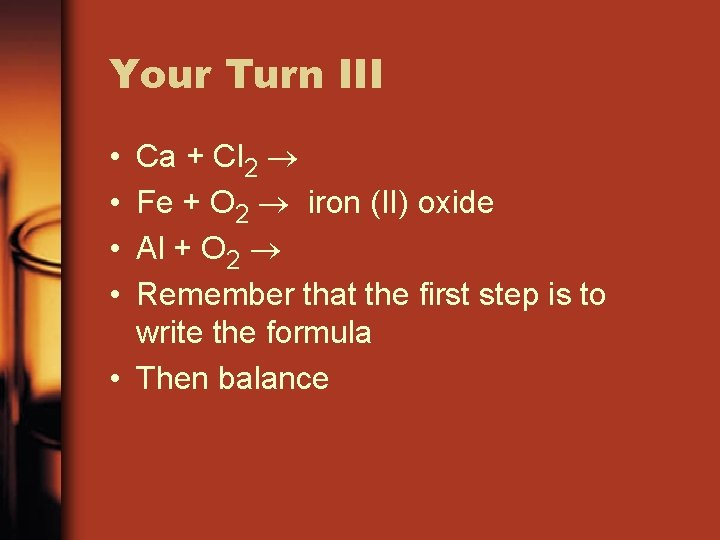Your Turn III Ca + Cl 2 Fe + O 2 iron (II) oxide Al + O 2 Remember that the first step is to write the formula • Then balance • •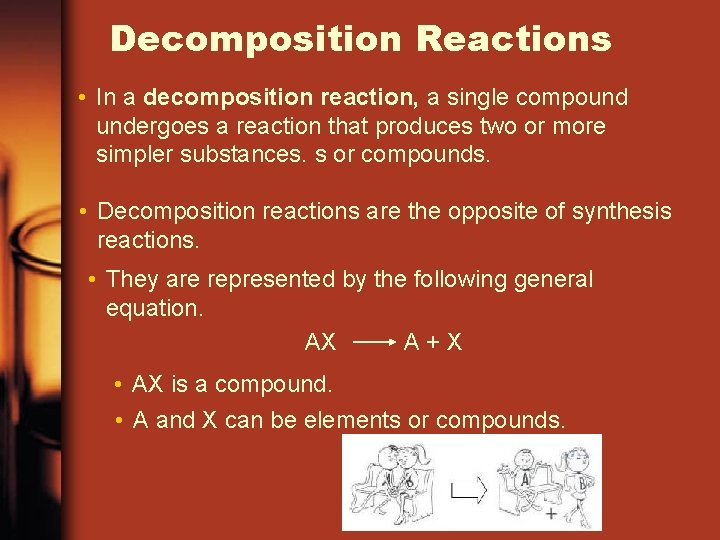Decomposition Reactions • In a decomposition reaction, a single compound undergoes a reaction that produces two or more simpler substances. s or compounds. • Decomposition reactions are the opposite of synthesis reactions. • They are represented by the following general equation. AX A+X • AX is a compound. • A and X can be elements or compounds.Decomposition of Binary Compounds • The decomposition of a substance by an electric current is called electrolysis. • example: • Oxides of the less-active metals, which are located in the lower center of the periodic table, decompose into their elements when heated. • example:Decomposition Reactions Decomposition of Metal Carbonates Decomposition of Metal Hydroxides Decomposition of Metal Chlorates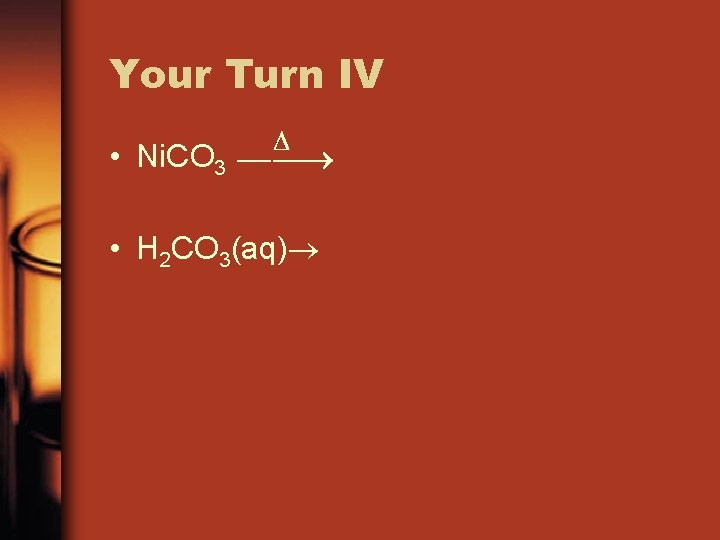Your Turn IV • Ni. CO 3 • H 2 CO 3(aq)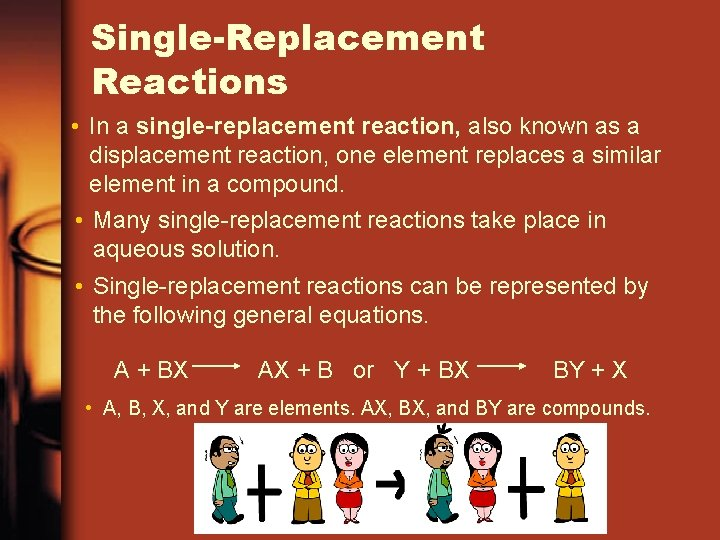Single-Replacement Reactions • In a single-replacement reaction, also known as a displacement reaction, one element replaces a similar element in a compound. • Many single-replacement reactions take place in aqueous solution. • Single-replacement reactions can be represented by the following general equations. A + BX AX + B or Y + BX BY + X • A, B, X, and Y are elements. AX, BX, and BY are compounds.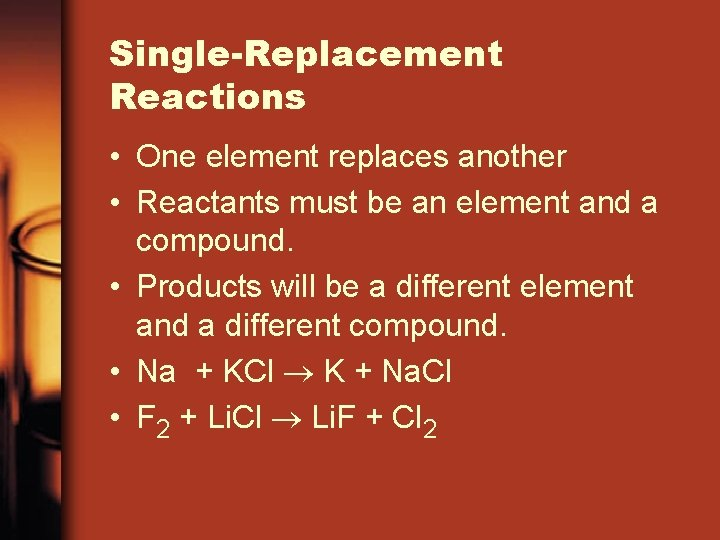Single-Replacement Reactions • One element replaces another • Reactants must be an element and a compound. • Products will be a different element and a different compound. • Na + KCl K + Na. Cl • F 2 + Li. Cl Li. F + Cl 2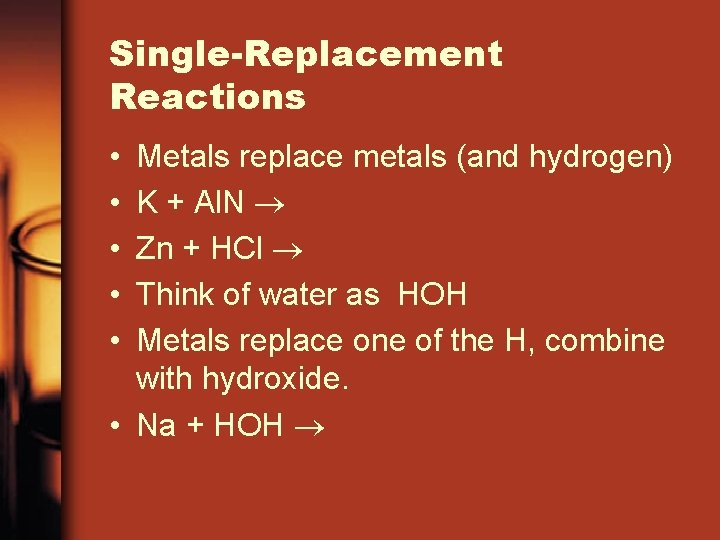Single-Replacement Reactions • • • Metals replace metals (and hydrogen) K + Al. N Zn + HCl Think of water as HOH Metals replace one of the H, combine with hydroxide. • Na + HOH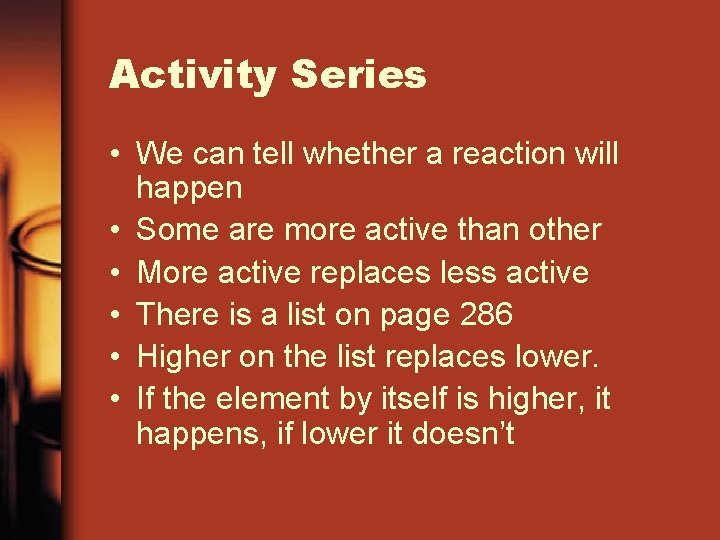Activity Series • We can tell whether a reaction will happen • Some are more active than other • More active replaces less active • There is a list on page 286 • Higher on the list replaces lower. • If the element by itself is higher, it happens, if lower it doesn’tReactivity SeriesActivity Series • H can be replaced in acids by everything higher • Only the first 6 (Li - Na) react with water. • Fe + Cu. SO 4 • Pb + KCl • Al + HClActivity Series • What does it mean that Au And Ag are on the bottom of the list? • Nonmetals can replace other nonmetals • Limited to F 2 , Cl 2 , Br 2 , I 2 • The order of activity is that on the table. • Higher replaces lower. • F 2 + HCl • Br 2 + KCl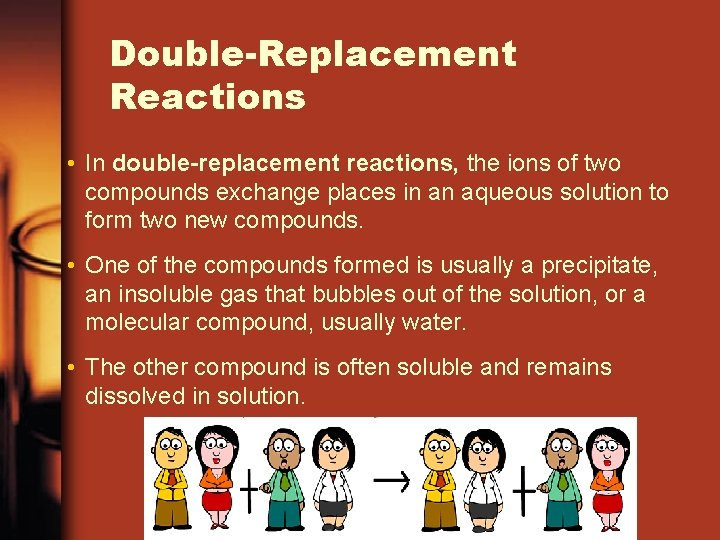Double-Replacement Reactions • In double-replacement reactions, the ions of two compounds exchange places in an aqueous solution to form two new compounds. • One of the compounds formed is usually a precipitate, an insoluble gas that bubbles out of the solution, or a molecular compound, usually water. • The other compound is often soluble and remains dissolved in solution.Double-Replacement Reactions • A double-displacement reaction is represented by the following general equation. AX + BY AY + BX • A, X, B, and Y in the reactants represent ions. • AY and BX represent ionic or molecular compounds.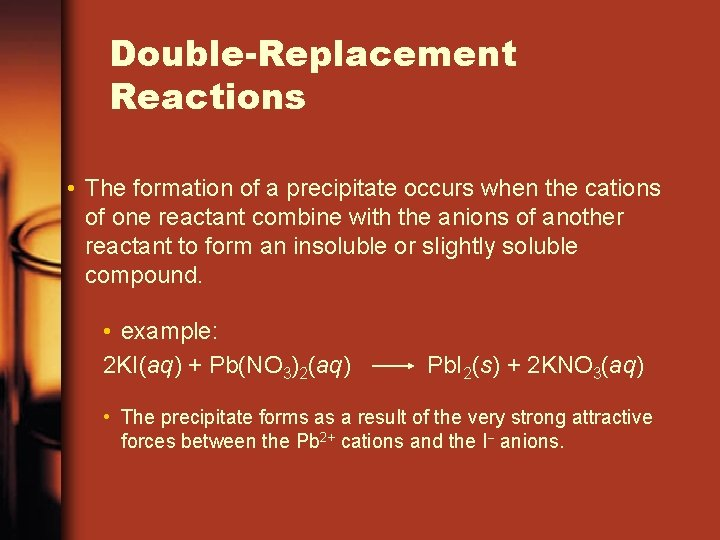Double-Replacement Reactions • The formation of a precipitate occurs when the cations of one reactant combine with the anions of another reactant to form an insoluble or slightly soluble compound. • example: 2 KI(aq) + Pb(NO 3)2(aq) Pb. I 2(s) + 2 KNO 3(aq) • The precipitate forms as a result of the very strong attractive forces between the Pb 2+ cations and the I anions.Double-Replacement Reactions • Two things replace each other. • Reactants must be two ionic compounds or acids. • Usually in aqueous solution • Na. OH + Fe. Cl 3 • The positive ions change place. • Na. OH + Fe. Cl 3 Fe+3 OH- + Na+1 Cl-1 • Na. OH + Fe. Cl 3 Fe(OH)3 + Na. ClDouble-Replacement Reactions • Will only happen if one of the products – doesn’t dissolve in water and forms a solid – or is a gas that bubbles out. – or is a covalent compound usually water.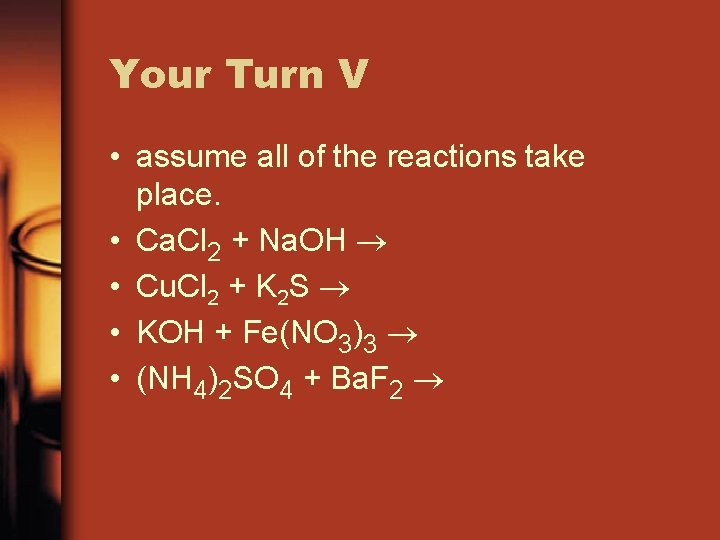Your Turn V • assume all of the reactions take place. • Ca. Cl 2 + Na. OH • Cu. Cl 2 + K 2 S • KOH + Fe(NO 3)3 • (NH 4)2 SO 4 + Ba. F 2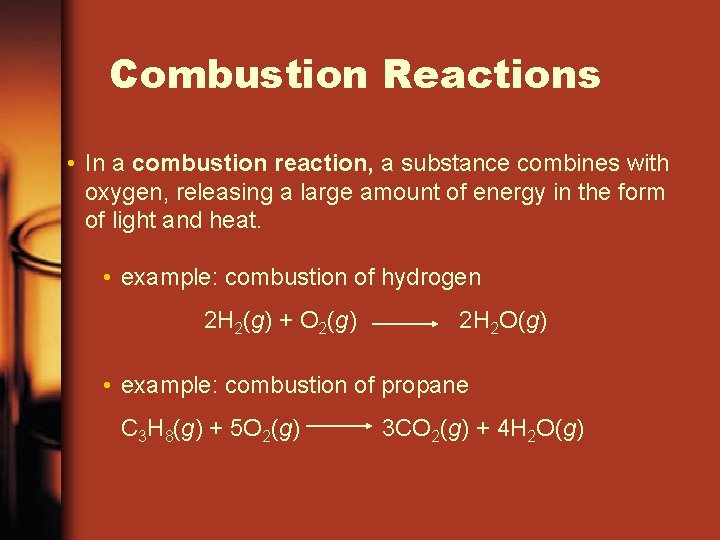Combustion Reactions • In a combustion reaction, a substance combines with oxygen, releasing a large amount of energy in the form of light and heat. • example: combustion of hydrogen 2 H 2(g) + O 2(g) 2 H 2 O(g) • example: combustion of propane C 3 H 8(g) + 5 O 2(g) 3 CO 2(g) + 4 H 2 O(g)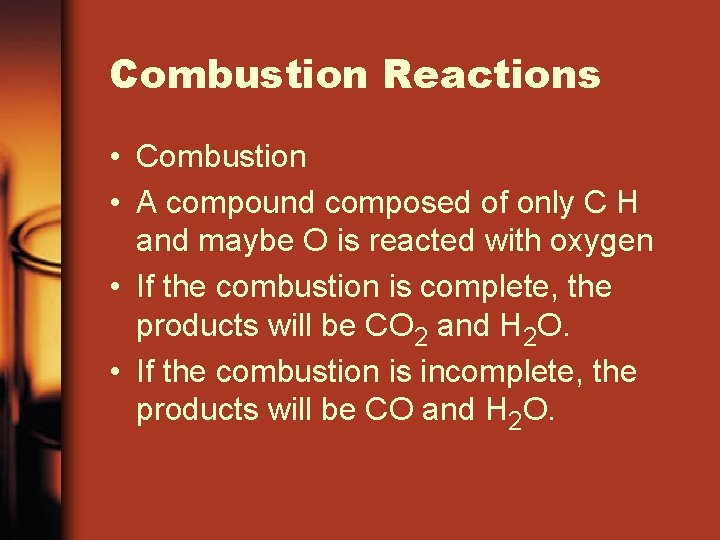Combustion Reactions • Combustion • A compound composed of only C H and maybe O is reacted with oxygen • If the combustion is complete, the products will be CO 2 and H 2 O. • If the combustion is incomplete, the products will be CO and H 2 O.Your Turn VI • C 4 H 10 + O 2 (complete) • C 4 H 10 + O 2 (incomplete) • C 6 H 12 O 6 + O 2 (complete) • C 8 H 8 +O 2 (incomplete)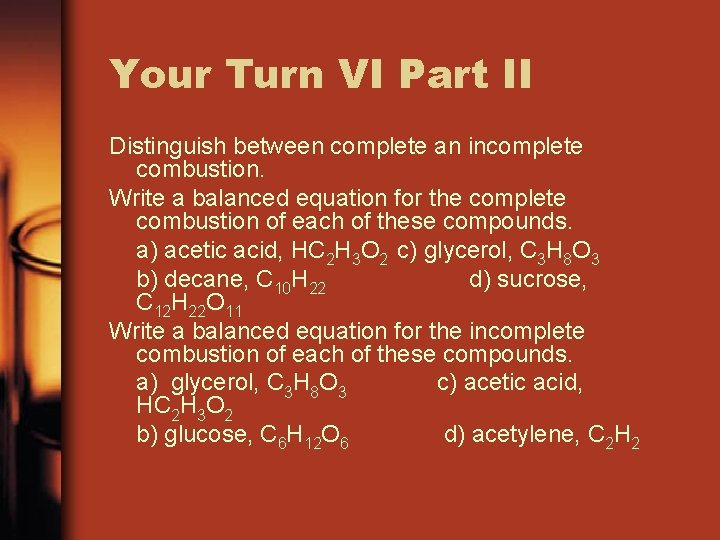Your Turn VI Part II Distinguish between complete an incomplete combustion. Write a balanced equation for the complete combustion of each of these compounds. a) acetic acid, HC 2 H 3 O 2 c) glycerol, C 3 H 8 O 3 b) decane, C 10 H 22 d) sucrose, C 12 H 22 O 11 Write a balanced equation for the incomplete combustion of each of these compounds. a) glycerol, C 3 H 8 O 3 c) acetic acid, HC 2 H 3 O 2 b) glucose, C 6 H 12 O 6 d) acetylene, C 2 H 2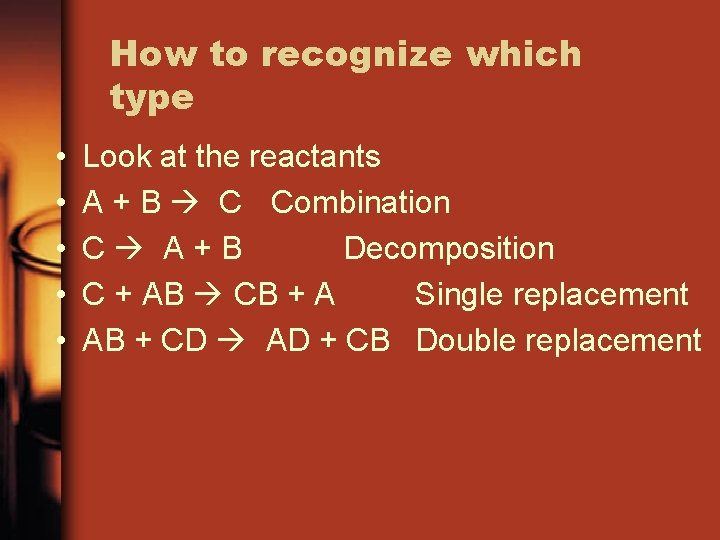How to recognize which type • • • Look at the reactants A + B C Combination C A+B Decomposition C + AB CB + A Single replacement AB + CD AD + CB Double replacement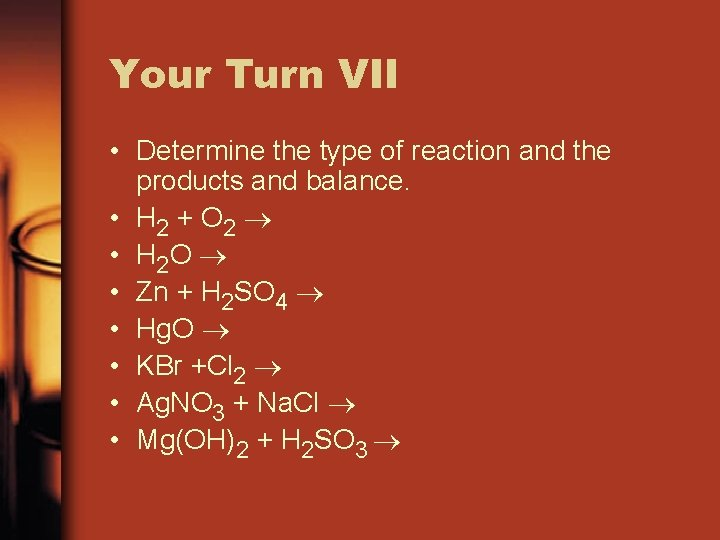Your Turn VII • Determine the type of reaction and the products and balance. • H 2 + O 2 • H 2 O • Zn + H 2 SO 4 • Hg. O • KBr +Cl 2 • Ag. NO 3 + Na. Cl • Mg(OH)2 + H 2 SO 3Let’s Review • Write out the balanced equation for Copper reacts with chlorine to form copper (II) chloride. • Write out the balanced equation for Zinc reacts with Hydrochloric acid to form Zinc chloride and hydrogen. • Write out the balanced equation for Calcium carbonate reacts with Zinc nitride to form Calcium nitride and Zinc carbonate.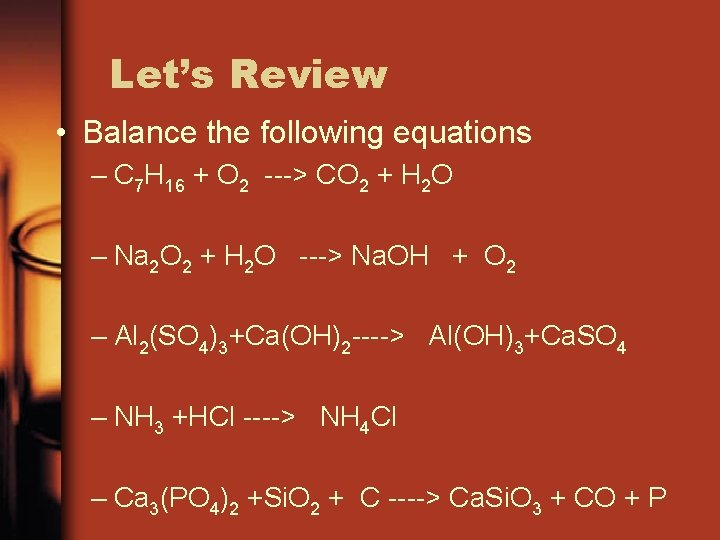Let’s Review • Balance the following equations – C 7 H 16 + O 2 ---> CO 2 + H 2 O – Na 2 O 2 + H 2 O ---> Na. OH + O 2 – Al 2(SO 4)3+Ca(OH)2 ----> Al(OH)3+Ca. SO 4 – NH 3 +HCl ----> NH 4 Cl – Ca 3(PO 4)2 +Si. O 2 + C ----> Ca. Si. O 3 + CO + P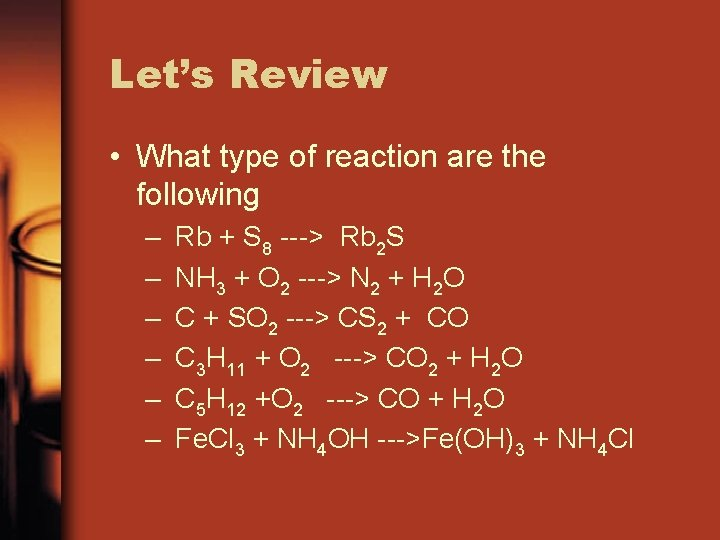Let’s Review • What type of reaction are the following – – – Rb + S 8 ---> Rb 2 S NH 3 + O 2 ---> N 2 + H 2 O C + SO 2 ---> CS 2 + CO C 3 H 11 + O 2 ---> CO 2 + H 2 O C 5 H 12 +O 2 ---> CO + H 2 O Fe. Cl 3 + NH 4 OH --->Fe(OH)3 + NH 4 Cl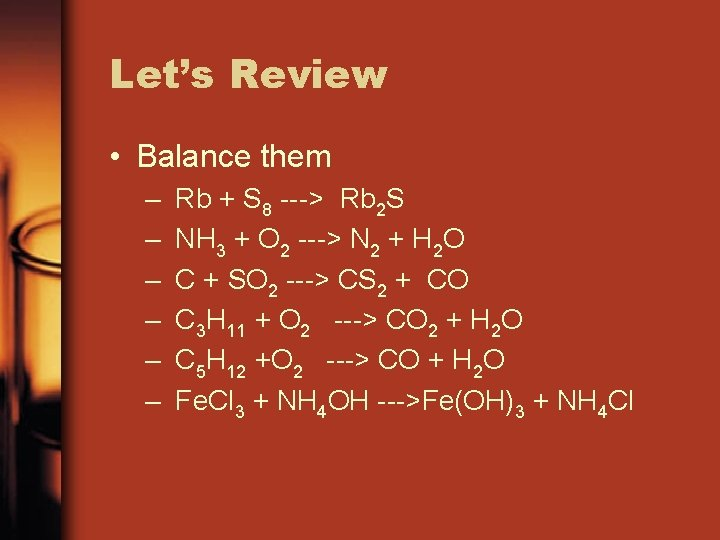Let’s Review • Balance them – – – Rb + S 8 ---> Rb 2 S NH 3 + O 2 ---> N 2 + H 2 O C + SO 2 ---> CS 2 + CO C 3 H 11 + O 2 ---> CO 2 + H 2 O C 5 H 12 +O 2 ---> CO + H 2 O Fe. Cl 3 + NH 4 OH --->Fe(OH)3 + NH 4 ClLet’s Review • Predict the products and balance – Sodium phosphate reacts with Lithium metal. – Butane (C 4 H 10) is completely combusted. – Sodium Chloride reacts with Silver Hydroxide – Aluminum Bromate reacts with Copper – Potassium reacts with Sulfur – Octane (C 8 H 18) is incompletely combusted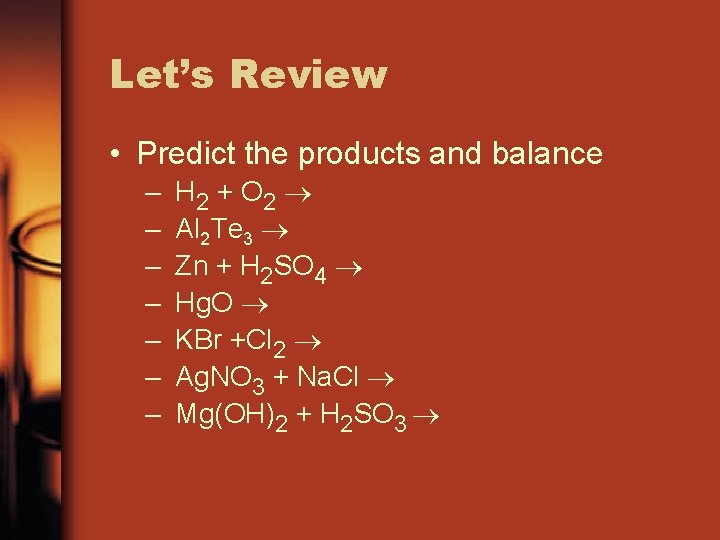Let’s Review • Predict the products and balance – – – – H 2 + O 2 Al 2 Te 3 Zn + H 2 SO 4 Hg. O KBr +Cl 2 Ag. NO 3 + Na. Cl Mg(OH)2 + H 2 SO 3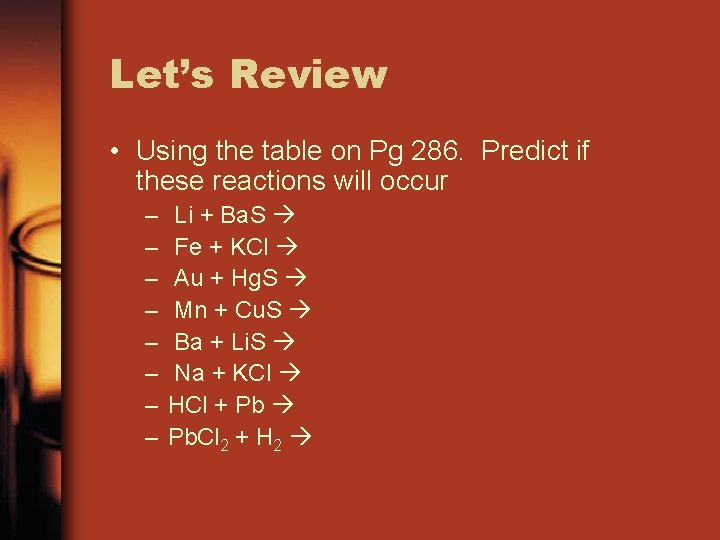Let’s Review • Using the table on Pg 286. Predict if these reactions will occur – – – – Li + Ba. S Fe + KCl Au + Hg. S Mn + Cu. S Ba + Li. S Na + KCl HCl + Pb Pb. Cl 2 + H 2